# Expressions with unknown variables 2

##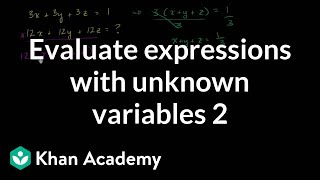By Khan Academy

A few more examples where Sal evaluates expressions with multiple unknown variables.# Expressions with unknown variables

##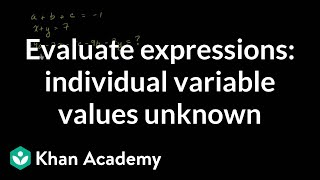By Khan Academy

Sal solved a few examples where we need to evaluate an expression, but we don't know the individual variable values.# Algebra Basics: What Is Algebra? - Math Antics

##By Lumos Learning

This video gives an overview of Algebra and introduces the concepts of unknown values and variables. It also explains that multiplication is implicit in Algebra.# Identifying the Equation and Solution to a Two-Step Problem: 3.OA.8

##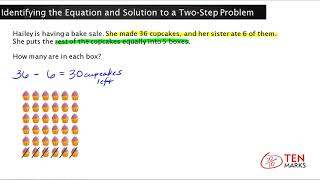By Tenmarks Amazon

Presents a two-step word problem involving one or two of the four operations. An equation is written with a variable for the unknown value.# Introduction to Random Variables - Khan Academy

##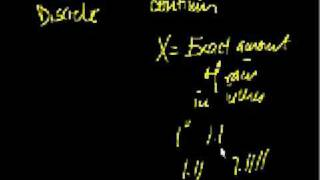By Khan Academy

This video lecture series from Khan Academy on Probability includes Introduction to Probability, Permutations, Combinations, Probability using Combinations, Conditional probability, Introduction to Random Variables, Probability Density Functions, Binomial Distribution, Expected Value, Expected value of Binomial Distribution, Poisson Process, Law of Large Numbers# Graphing - Independent Dependent Variables

##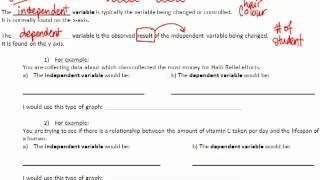By MrG Lewis

Learn how to graph independent and dependent variables in this video from Mr G Lewis# Expressions and variables

##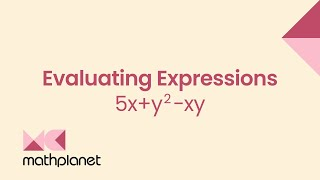By MathPlanetVideos

Evaluate the expression when x=4 and y=3 5x+y2−xy# Mean and Expected Value of Discrete Random Variables

##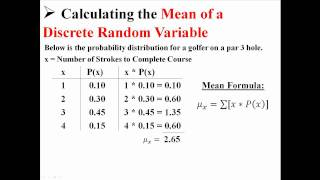By statslectures

This video summarizes for us in less than two minutes how to find the expected value for a discrete random variable. In order to apply this to empirical data, form a histogram from your data and use that to determine relative frequencies.# Example 3: Finding the square of a binomial with two variables | Algebra I | Khan Academy

##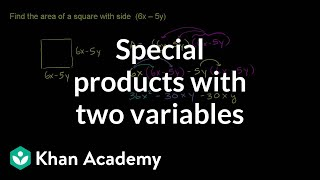By Khan Academy

This Khan Academy video offers more information on special products and contains a few samples with a step-by-step walkthrough on these new concepts.# Example 6: Subtracting polynomials with two variables | Algebra I | Khan Academy

##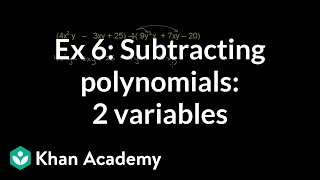By Khan Academy

Example 6: Subtracting polynomials with two variables | Algebra I | Khan Academy# Independent and Dependent Variables - YourTeacher.com

##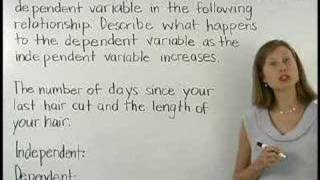By yourteachermathhelp

This video briefly explains the difference between an independent and dependent variable using the example of the length of your hair based on how many days it has been since you got a hair cut.# Example 3: Finding the square of a binomial with two variables | Algebra I | Khan Academy

##By Khan Academy

This Khan Academy video offers more information on special products and contains a few samples with a step-by-step walkthrough on these new concepts.# Example 6: Factoring a difference of squares with two variables | Algebra II | Khan Academy

##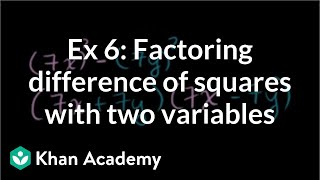By Khan Academy

Khan Academy presents Example 2: Factoring difference of squares, an educational video resource on math.# Evaluating Expressions with Two Variables - Khan Academy

##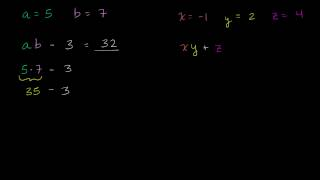By Khan Academy

Sal Khan shows the viewer how to evaluate an expression with two variables.# Linear Equations in Two Variables

##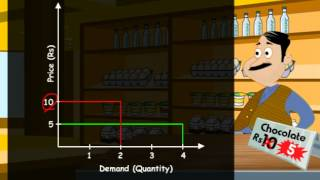By Meritnation

YouTube presents Linear Equations in Two Variables, an educational video resource on math.# Solving systems of equations in three variables

##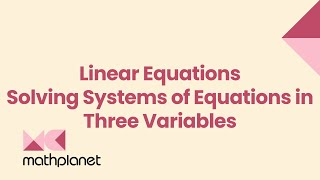By MathPlanetVideos

Solve the systems of equation in our example.# Equations with variables

##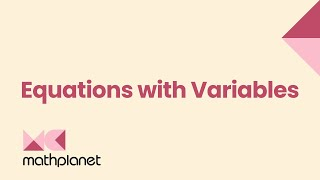By MathPlanetVideos

Solve the following equation 8⋅x−x=21# Solving systems of equations in two variables

##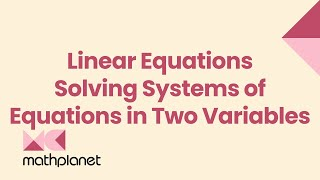By MathPlanetVideos

Solve the system of equations: {2x−4y=0 −4x+4y=−4# Add and subtract polynomials with two variables

##By Khan Academy

Sal simplifies (4x^2y - 3xy + 25) - (9y^2x + 7xy - 20).# Mean E(X) and Variance Var(X) for Continuous Random Variables : ExamSolutions

##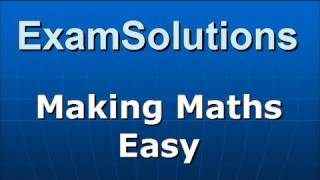By ExamSolutions

If we took the same approach to finding the expected value for a continuous random variable as we took for a discrete random variable, we would have to sum up an infinite number of terms. Fortunately, we have the field of calculus to help us with what would otherwise be an insurmountable task. This video shows us how we can use integrals to find the expected value of a continuous random variable. This video also goes over finding the variance of a continuous random variable, which requires the use of the expected value itself. The basic skill set is the same, though, so it might be valuable to watch as well.